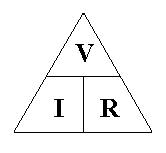# Ohms Law

This simple formula describes the relationship between voltage, current and resistance:

Voltage = Current X Resistance or V = IR

Current = Voltage / Resistance or I = V / R

Resistance = Voltage / Current or R = V / I

Ohms law can easily be remembered using the triangle below. Cover Up the value you want to find, and treat letters next to each other  as multiplications, and letters above each other as divisions. Try it!